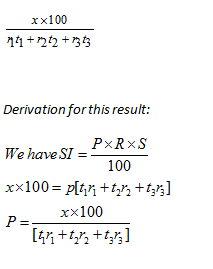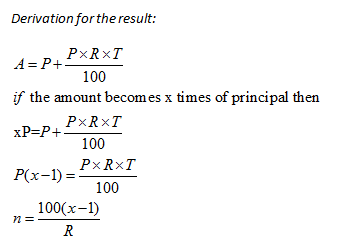Tips, Tricks & Results for Simple Interest-1

The purpose of this article is to provide you with some useful tips and tricks that you can use for Simple Interest questions. Various applications and formulas based on this concept are explained here.

Simple Interest Tips, Tricks, and Results: Tooltip 1

If the interest on a sum of money is 1/x  of the principal, and the number of years is equal to the rate of interest then rate can be calculated using the formula: √(100/x)

Derivation for this result:
We Know SI = {(P x R x T)/100}
Put SI = P/x  ; and T=R
P/x = {(P x R x T)/100}
R2 = 100/x
R = √(100/x)

Example : The interest on a sum of money is 1/16 of the principal, and the number of years is equal to the rate of interest. What is the rate percent?

Solution :

Using the above concept :

R = √(100/x)

R = √(100/16)

R = 5/2%

Simple Interest Tips, Tricks and Results: Tooltip 2

The rate of interest for t1 years is r1% , t2 years is r1%, t2 years is r3 %.If a man gets interest of Rs x

for (t1+ t2 + t3 =n)  years, then principal is given byExample: The rate of interest for 3 years is 4%, 5years is 6%,1 years is 5%. If a man gets interest of Rs. 4700 for 9 years, calculate the principal amount?Example: A sum of money becomes 4 times in 20 years. Calculate the rate of interest.Example: A sum of money becomes 4 times in 20 years. Calculate the rate of interest.

Solution:

Using the above result:

R = [{100(x-1)}/n]%

R = [{100(4-1)}/20]=15%

Simple Interest Tips, Tricks, and Results: Tooltip 4

If a sum of money becomes x times in n years at simple rate of interest, then the time is calculated as

n= {100(x-1)/R}Example:A sum was put at SI at a certain rate for 3 years. If the sum would have put at a 2% higher rate, it would have yieldedRs 900 more. What will be the principal?

Solution:

Use the above formula:

P= {100X/(nX r)}

P= {(900 x 100)/(3 x2)} = Rs. 15000

This tooltip is a reverse of the last one. A sum is put at SI at a certain rate for n years. If the sum would have put at a r% lower rate, it could yield Rs X less. So the principal will be:

P= {100X/(n * r)}

Example: A sum was put at SI at a certain rate for 3 years. If the sum would have put at a 2% lower rate, it could yield Rs 900 less. What will be the principal?

Solution:

Using the formula, we arrive at the following:

P= {100X/(n * r)}

P= {(900*100)/(3*2)}

P = Rs. 15000